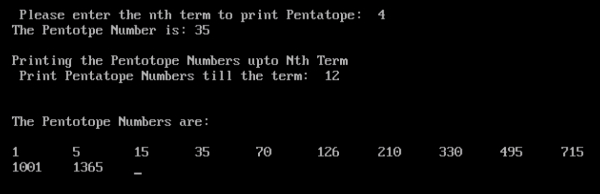# Program to print pentatope numbers upto Nth term in C

## Program Description

A pentatope number is a number in the fifth cell of any row of Pascal's triangle starting with the 5-term row 1 4 6 4 1 either from left to right or from right to left.

The first few numbers of this kind are

1, 5, 15, 35, 70, 126, 210, 330, 495, 715, 1001, 1365

Pentatope numbers belong in the class of figurate numbers, which can be represented as regular, discrete geometric patterns. The formula for the nth pentatopic number is

$$\left(\begin{array}{c}n+3\ 4\end{array}\right)=\left(\frac{n(n+1)+(n+2)+(n+3)}{24}\right)=\left(\frac{n^2}{4!}\right)$$

## Algorithm

Accept the Nth Term from the User to find the Pentotope Numbers.

Use the formula

$$\left(\begin{array}{c}n+3\ 4\end{array}\right)=\left(\frac{n(n+1)+(n+2)+(n+3)}{24}\right)=\left(\frac{n^2}{4!}\right)$$

## Example

/* Program to print pentatope numbers upto Nth term */
#include<stdio.h>
int main() {
int n, n1, nthterm, nthterm1, i;
clrscr();
printf(" Please enter the nth term to print Pentatope: ");
scanf("%d",&n);
nthterm = n * (n + 1) * (n + 2) * (n + 3) / 24;
printf("The Pentotpe Number is: ");
printf("%d", nthterm);
printf("");
printf("Printing the Pentotope Numbers upto Nth Term");
printf(" Print Pentatope Numbers till the term: ");
scanf("%d",&n1);
printf("");
printf("The Pentotope Numbers are:");
printf("");
for (i = 1; i <= n1; i++){
nthterm1 = (i * (i + 1) * (i + 2) * (i + 3) / 24);
printf("%d\t", nthterm1);
}
getch();
return 0;
}

## Output